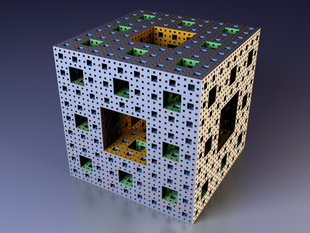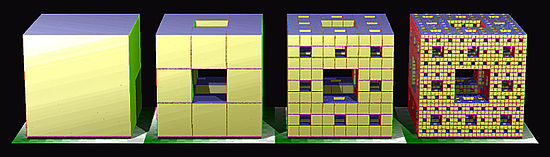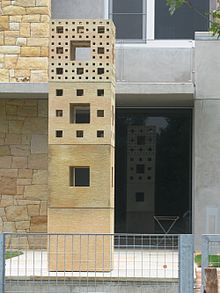# Menger sponge

﻿
Menger spongeAn illustration of M4, the fourth iteration of the construction process.

In mathematics, the Menger sponge is a fractal curve. It is a universal curve, in that it has topological dimension one, and any other curve (more precisely: any compact metric space of topological dimension 1) is homeomorphic to some subset of it. It is sometimes called the Menger-Sierpinski sponge or the Sierpinski sponge. It is a three-dimensional extension of the Cantor set and Sierpinski carpet. It was first described by Karl Menger (1926) while exploring the concept of topological dimension.

The Menger sponge simultaneously exhibits an infinite surface area and encloses zero volume.

## Construction

The construction of a Menger sponge can be described as follows:

1. Begin with a cube (first image).
2. Divide every face of the cube into 9 squares, like a Rubik's Cube. This will sub-divide the cube into 27 smaller cubes.
3. Remove the smaller cube in the middle of each face, and remove the smaller cube in the very center of the larger cube, leaving 20 smaller cubes (second image). This is a level-1 Menger sponge (resembling a Void Cube).
4. Repeat steps 2 and 3 for each of the remaining smaller cubes, and continue to iterate ad infinitum.

The second iteration will give you a level-2 sponge (third image), the third iteration gives a level-3 sponge (fourth image), and so on. The Menger sponge itself is the limit of this process after an infinite number of iterations.An illustration of the iterative construction of a Menger sponge up to M3, the third iteration.

The number of cubes is 20n, with n being the number of iterations performed on the first cube.A sculptural representation of the previous illustration.

## Properties

Each face of the Menger sponge is a Sierpinski carpet; furthermore, any intersection of the Menger sponge with a diagonal or medium of the initial cube M0 is a Cantor set.

The Menger sponge is a closed set; since it is also bounded, the Heine–Borel theorem implies that it is compact. It has Lebesgue measure 0. It is an uncountable set.

The topological dimension of the Menger sponge is one, the same as any curve. Menger showed, in the 1926 construction, that the sponge is a universal curve, in that any possible one-dimensional curve is homeomorphic to a subset of the Menger sponge, where here a curve means any compact metric space of Lebesgue covering dimension one; this includes trees and graphs with an arbitrary countable number of edges, vertices and closed loops, connected in arbitrary ways. In a similar way, the Sierpinski carpet is a universal curve for all curves that can be drawn on the two-dimensional plane. The Menger sponge constructed in three dimensions extends this idea to graphs that are not planar, and might be embedded in any number of dimensions.

The Menger sponge simultaneously exhibits an infinite surface area and encloses zero volume. In spite of this, there exists a homeomorphism of the cube having finite distortion that "squeezes the sponge" in the sense that the holes in the sponge go to a Cantor set of zero measure (Iwaniec & Martin 2001, §6.5.6).

The sponge has a Hausdorff dimension of (log 20) / (log 3) (approximately 2.726833).

## Formal definition

Formally, a Menger sponge can be defined as follows:$M := \bigcap_{n\in\mathbb{N}} M_n$

where M0 is the unit cube and$M_{n+1} := \left\{\begin{matrix} (x,y,z)\in\mathbb{R}^3: & \begin{matrix}\exists i,j,k\in\{0,1,2\}: (3x-i,3y-j,3z-k)\in M_n \\ \mbox{and at most one of }i,j,k\mbox{ is equal to 1}\end{matrix} \end{matrix}\right\}.$

Wikimedia Foundation. 2010.

### Look at other dictionaries:

• Menger-Schwamm — nach der 4. Iterationsstufe Der Menger Schwamm gehört wie das Sierpinski Dreieck und die Koch Kurve zu den Objekten der fraktalen Geometrie. Der nach Karl Menger benannte Schwamm wurde zum ersten Mal 1926 in seiner Arbeit über Dimensionalität von …   Deutsch Wikipedia

• Menger — may refer to: People Andreas Menger (born 1972), former German football player Anton Menger (1841–1906), Austrian economist and author; brother of Carl Menger Carl Menger (1840–1921), Austrian economist and author founder of the Austrian School… …   Wikipedia

• Sponge (disambiguation) — Sponge may refer to:* Sponges, animals of the phylum Porifera * Sponge (tool), a tool consisting of porous material used for cleaning impervious surfaces ** Sponge rubber tube cleaning ball, serves for tube cleaning in the so called Taprogge… …   Wikipedia

• Karl Menger — This article is about the mathematician (who also contributed to economics), not about his father, the economist Carl Menger. Karl Menger (Vienna, Austria, January 13 1902 – Highland Park, Illinois, U.S., October 5 1985) was a mathematician of… …   Wikipedia

• Institute For Figuring — The Institute For Figuring (IFF) is an organization based in Los Angeles, California that promotes the public understanding of the poetic and aesthetic dimensions of science, mathematics and the technical arts. The Institute hosts public lectures …   Wikipedia

• Silk (2006 film) — Infobox Film name =Silk imagesize =282 px imagecaption = imdb id =0494834 writer = Chao Bin Su starring =Chang Chen Chun Ning Chang Bo lin Chen Yosuke Eguchi Barbie Hsu director =Chao Bin Su distributor =CMC Entertainment released = September 28 …   Wikipedia

• n-flake — An n flake, polyflake, or Sierpinski n gon, is a fractal constructed starting from an n gon. This n gon is replaced by a flake of smaller n gons, such that the scaled polygons are placed at the vertices, and sometimes in the center. This… …   Wikipedia

• Mengerscher Schwamm — Der Menger Schwamm (nach Karl Menger benannt) gehört wie das Sierpinski Dreieck und die Koch Kurve zu den Objekten der fraktalen Geometrie. Der Mengersche Schwamm ist ein dreidimensionales Analogon der Cantor Menge oder des Sierpinski Teppichs:… …   Deutsch Wikipedia

• Cantor set — In mathematics, the Cantor set, introduced by German mathematician Georg Cantor in 1883 [Georg Cantor (1883) Über unendliche, lineare Punktmannigfaltigkeiten V [On infinite, linear point manifolds (sets)] , Mathematische Annalen , vol. 21, pages… …   Wikipedia

• Fractal — A fractal is generally a rough or fragmented geometric shape that can be split into parts, each of which is (at least approximately) a reduced size copy of the whole, [cite book last = Mandelbrot first = B.B. title = The Fractal Geometry of… …   Wikipedia